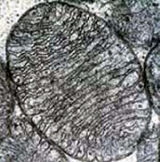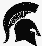xSurface area
Posts  1 - 2  of  2NURSE46
WHATS THE DIFFERENCE BETWEEN SURFACE AREA AND VOLUME, AND WHAT ARE THEY SIMILARITIES
replied to:  NURSE46AllSmiles
Replied to:  WHATS THE DIFFERENCE BETWEEN SURFACE AREA AND VOLUME, AND WHAT ARE...
Surface area is the measure of how much exposed area a solid object has, expressed in square units. Mathematical description of the surface area is considerably more involved than the definition of arc length.
Arc length
Determining the length of an irregular arc segment is also called rectification of a curve. Historically, many methods were used for specific curves...
of a curve. For polyhedra (objects with flat polygonal faces
Face (geometry)
In geometry, a face of a polyhedron is any of the polygons that make up its boundaries. For example, any of the squares that bound a cube is a face of the cube. The suffix -hedron is derived from the Greek word hedra which means face....
) the surface area is the sum of the areas of its faces. Smooth surfaces, such as a sphere
Sphere
A sphere is a perfectly round geometrical object in three-dimensional space, such as the shape of a round ball. Like a circle in two dimensions, a perfect sphere is completely symmetrical around its center, with all points on the surface lying the same distance r from the center point...
, are assigned surface area using their representation as parametric surface
Parametric surface
A parametric surface is a surface in the Euclidean space R3 which is defined by a parametric equation with two parameters. Parametric representation is the most general way to specify a surface. Surfaces that occur in two of the main theorems of vector calculus, Stokes' theorem and the...
s.
Volume is how much three-dimensional space a substance (solid, liquid, gas, or plasma) or shape occupies or contains,often quantified numerically using the SI derived unit, the cubic metre. The volume of a container is generally understood to be the capacity of the container, i. e. the amount of fluid (gas or liquid) that the container could hold, rather than the amount of space the container itself displaces.
Three dimensional mathematical shapes are also assigned volumes. Volumes of some simple shapes, such as regular, straight-edged, and circular shapes can be easily calculated using arithmetic formulas. The volumes of more complicated shapes can be calculated by integral calculus if a formula exists for the shape's boundary. One-dimensional figures (such as lines) and two-dimensional shapes (such as squares) are assigned zero volume in the three-dimensional space.